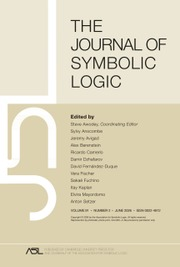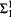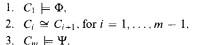Skip to main content Accessibility help
Home
Hostname: page-component-5bf98f6d76-rs6k2 Total loading time: 0.488 Render date: 2021-04-21T01:55:55.807Z Has data issue: true Feature Flags: { "shouldUseShareProductTool": true, "shouldUseHypothesis": true, "isUnsiloEnabled": true, "metricsAbstractViews": false, "figures": false, "newCiteModal": false, "newCitedByModal": true }The Journal of Symbolic Logic

# A form of feasible interpolation for constant depth Frege systems

Published online by Cambridge University Press:  12 March 2014

Corresponding
E-mail address:

## Abstract

Let L be a first-order language and Φ and Ψ twoL-sentences that cannot be satisfied simultaneously in any finite L-structure. Then obviously the following principle ChainL,Φ,Ψ(n, m) holds: For any chain of finite L-structures C1, …, Cm with the universe [n] one of the following conditions must fail:For each fixed L and parameters n, m the principle ChainL,Φ,Ψ(n,m) can be encoded into a propositional DNF formula of size polynomial in n, m.

For any language L containing only constants and unary predicates we show that there is a constant CL such that the following holds: If a constant depth Frege system in DeMorgan language proves ChainL,Φ,Ψ(n, cL . n) by a size s proof then the class of finite L-structures with universe [n] satisfying Φ can be separated from the class of those L-structures on [n] satisfying ψ by a depth 3 formula of size 2log(S)O(1) and with bottom fan-in log(S)O(1).

Type
Research Article
Information
The Journal of Symbolic Logic , June 2010 , pp. 774 - 784
Copyright
Copyright © Association for Symbolic Logic 2010

## Access options

Get access to the full version of this content by using one of the access options below.

## References

Ajtai, M., The complexity of the pigeonhole principle. Proceedings of the IEEE Annual Symposium on Foundations of Computer Science (FOCS), 1988, pp. 346355.Google Scholar
Bonet, M. L., Domingo, C., Gavalda, R., Maciel, A., and Pitassi, T., Non-automatizability of bounded-depth Frege proofs, Computational Complexity, vol. 13 (2004), pp. 4768.CrossRefGoogle Scholar
Bonet, M. L., Pitassi, T., and Raz, R., Lower bounds for cutting planes proofs with small coefficients, this Journal, (1997), pp. 708728.Google Scholar
Bonet, M. L., On interpolation and automatization for Frege systems, SIAM Journal on Computing, vol. 29 (2000), no. 6, pp. 19391967.CrossRefGoogle Scholar
Buss, S. R., Bounded Arithmetic, Naples, Bibliopolis, 1986.Google Scholar
Buss, S. R., The prepositional pigeonhole principle has polynomial size Frege proofs, this Journal, vol. 52 (1987), pp. 916927.Google Scholar
Cook, S. A. and Nguyen, P., Logical foundations of proof complexity, Perspectives in Logic, Cambridge University Press, 2010.CrossRefGoogle Scholar
Cook, S. A. and Reckhow, A. R., The relative efficiency of prepositional proof systems, this Journal, vol. 44 (1979), no. 1, pp. 3650.Google Scholar
Hastad, J., Almost optimal lower bounds for small depth circuits, Randomness and Computation (Micali, S., editor), Advances in Computing Research, vol. 5, JAI Press, 1989, pp. 143170.Google Scholar
Krajíček, J., Lower bounds to the size of constant-depth prepositional proofs, this Journal, vol. 59 (1994), no. 1, pp. 7386.Google Scholar
Krajíček, J., Bounded arithmetic, prepositional logic, and complexity theory, Encyclopedia of Mathematics and Its Applications, vol. 60, Cambridge University Press, 1995.CrossRefGoogle Scholar
Krajíček, J., Interpolation theorems, lower bounds for proof systems, and independence results for bounded arithmetic, this Journal, vol. 62 (1997), no. 2, pp. 457486.Google Scholar
Krajíček, J., Discretely ordered modules as a first-order extension of the cutting planes proof system, this Journal, vol. 63 (1998), no. 4, pp. 15821596.Google Scholar
Krajíček, J., An exponential lower bound for a constraint propagation proof system based on ordered binary decision diagrams, this Journal, vol. 73 (2008), no. 1, pp. 227237.Google Scholar
Krajíček, J. and Pudlák, P., Some consequences of cryptographical conjectures forand EF, Logic and Computational Complexity (Proceedings of the Meeting held in Indianapolis, October 1994) (Leivant, D., editor), Lecture Notes in Computer Science, vol. 960, Springer-Verlag, 1995, Revised version in: Information and Computation, 140 (1998), no. 1, pp. 82–94, pp. 210220.Google Scholar
Krajíček, J., P. Pudlák, and Woods, A., An exponential lower bound to the size of bounded depth Frege proofs of the pigeonhole, Random Structures and Algorithms, vol. 7 (1995), no. 1, pp. 1539.CrossRefGoogle Scholar
Paris, J. B. and Wilkie, A. J., Counting problems in bounded arithmetic, Methods in Mathematical Logic, Lecture Notes in Mathematics, vol. 1130, Springer-Verlag, 1985, pp. 317340.CrossRefGoogle Scholar
Paris, J. B., Wilkie, A. J., and Woods, A. R., Provability of the pigeonhole principle and the existence of infinitely many primes, this Journal, vol. 53 (1988), pp. 12351244.Google Scholar
Pitassi, T., Beame, P., and Impagliazzo, R., Exponential lower bounds for the pigeonhole principle, Computational Complexity, vol. 3 (1993), pp. 97308.CrossRefGoogle Scholar
Pudlák, P., Lower bounds for resolution and cutting plane proofs and monotone computations, this Journal, (1997), pp. 981998.Google Scholar
Pudlák, P., The lengths of proofs, Handbook of Proof Theory (Buss, S. R., editor), Elsevier, 1998, pp. 547637.CrossRefGoogle Scholar
Pudlák, P. and Sgall, J., Algebraic models of computation and interpolation for algebraic proof systems, Proof Complexity and Feasible Arithmetic (Beame, P. and Buss, S. R., editors), DIMACS Series in Discrete Mathematics and Computer Science, vol. 39, American Mathematical Society, 1998, pp. 179205.Google Scholar
Razborov, A. A., Unprovability of lower bounds on the circuit size in certain fragments of bounded arithmetic, Izvestiya: Mathematics, vol. 59 (1995), no. 1, pp. 205227.CrossRefGoogle Scholar
Skelley, A. and Thapen, N., The provably total search problems of bounded arithmetic, 2008, Submitted.Google Scholar
Yao, A., Separating the polynomial-time hierarchy by oracles, Proceedings of the IEEE Annual Symposium on Foundations of Computer Science (FOCS), 1985, pp. 110.Google Scholar

### Full text views

Full text views reflects PDF downloads, PDFs sent to Google Drive, Dropbox and Kindle and HTML full text views.

Total number of HTML views: 0
Total number of PDF views: 13 *
View data table for this chart

* Views captured on Cambridge Core between September 2016 - 21st April 2021. This data will be updated every 24 hours.

# Send article to Kindle

To send this article to your Kindle, first ensure no-reply@cambridge.org is added to your Approved Personal Document E-mail List under your Personal Document Settings on the Manage Your Content and Devices page of your Amazon account. Then enter the ‘name’ part of your Kindle email address below. Find out more about sending to your Kindle. Find out more about sending to your Kindle.

Note you can select to send to either the @free.kindle.com or @kindle.com variations. ‘@free.kindle.com’ emails are free but can only be sent to your device when it is connected to wi-fi. ‘@kindle.com’ emails can be delivered even when you are not connected to wi-fi, but note that service fees apply.

Find out more about the Kindle Personal Document Service.

A form of feasible interpolation for constant depth Frege systems
Available formats
×

# Send article to Dropbox

To send this article to your Dropbox account, please select one or more formats and confirm that you agree to abide by our usage policies. If this is the first time you use this feature, you will be asked to authorise Cambridge Core to connect with your <service> account. Find out more about sending content to Dropbox.

A form of feasible interpolation for constant depth Frege systems
Available formats
×

# Send article to Google Drive

To send this article to your Google Drive account, please select one or more formats and confirm that you agree to abide by our usage policies. If this is the first time you use this feature, you will be asked to authorise Cambridge Core to connect with your <service> account. Find out more about sending content to Google Drive.

A form of feasible interpolation for constant depth Frege systems
Available formats
×
×

#### Conflicting interests

Do you have any conflicting interests? *